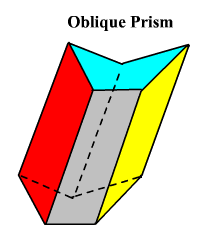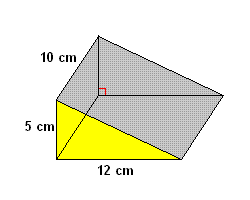# Prism

A prism is a three-dimensional figure or polyhedron having two faces (called the bases of the prism) which are congruent polygons, and the remaining faces parallelograms .If the remaining faces are rectangles (as in the figure $2$ nd figure above), then the prism is called a right prism . School level math books usually only talk about right prisms, and sometimes when they say prism they mean right prism.

A triangular prism is a prism with a triangular base, like the figure on the right. A rectangular prism is a prism with a rectangular base. (The cube is a special case.) Similarly, a prism with a $5$ -sided base is a pentagonal prism , a prism with a $6$ -sided base is a hexagonal prism , etc.

### Volume of a Prism

The volume $V$ of a right prism is given by the formula

$V=Bh$ ,

where $B$ is the area of the base and $h$ is the height.

Example:

Find the volume of the prism shown.The height of the prism is $10$ cm. The base is a right triangle with legs of length $5$ cm and $12$ cm, so

Therefore, the volume is

Note that for a rectangular prism, the volume formula becomes simply

$V=lwh$ ,

where $l$ is the length of the base, $w$ is the width of the base, and $h$ is the height of the prism.

### Surface Area of a Prism

The surface area $S$ of a prism is given by the formula

$S=2B+Ph$ ,

where $B$ is the area of the base of the prism, $P$ is the perimeter of the base, and $h$ is the height.

In the example above, by the Pythagorean theorem , the hypotenuse of the triangular base has length

So the perimeter of the base is $5+12+13=30$ cm. We already know the base area is $30$ cm 2 . So,

You may sometimes be called upon to find the lateral surface area of a prism. This just means the surface area of the faces not including the bases, or $L=Ph$ .